# How to read a metric micrometer?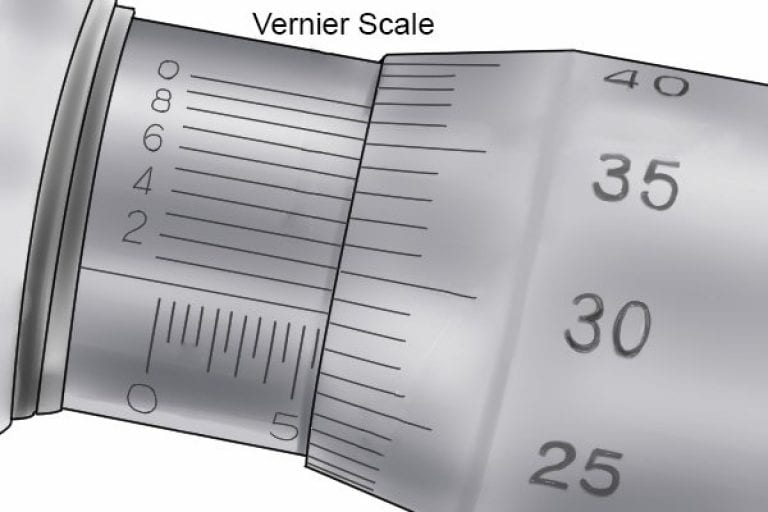Measurements taken with micrometer are a combination of the value shown on the sleeve scale and the value indicated on the rotating sleeve scale.

When using a micrometer with an additional vernier scale, the value on the vernier scale may also be taken into account.

## Guide to Reading a Metric Micrometer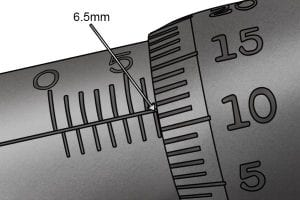Step 1 – Look at Sleeve Scale

The value of the first significant figure of a measurement can be found on the sleeve scale.

This will be the number immediately to the left of the thimble.

On a metric micrometer, this will be given in millimetres. Each minor increment on the sleeve scale represents 0.5mm.

In this example, the reading on the sleeve scale is 6.5mm.

Step 1 – Look at Sleeve Scale

The value of the first significant figure of a measurement can be found on the sleeve scale.

This will be the number immediately to the left of the thimble.

On a metric micrometer, this will be given in millimetres. Each minor increment on the sleeve scale represents 0.5mm.

In this example, the reading on the sleeve scale is 6.5mm.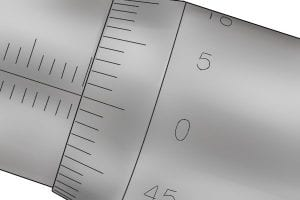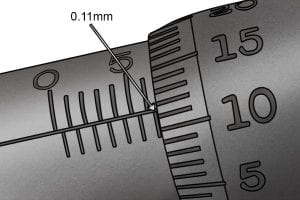Step 2 – Look at the Thimble Scale

Then, read the value shown on the thimble scale.

The thimble scale of a metric micrometer has a range of 0.5mm (one complete revolution), with each increment representing 0.01mm, and usually numbered in 0.05mm steps. The value is the lowest number that aligns best with the index line on the sleeve scale.

If the index line falls between

two values, the reading will be the number immediately below the index line.

If this is the case, you should estimate the final part of the measurement or, if your micrometer has one, consult the vernier scale.

Here, the thimble scale is showing 0.11mm.

Step 2 – Look at the Thimble Scale

Then, read the value shown on the thimble scale.

The thimble scale of a metric micrometer has a range of 0.5mm (one complete revolution), with each increment representing 0.01mm, and usually numbered in 0.05mm steps. The value is the lowest number that aligns best with the index line on the sleeve scale.If the index line falls between two values, the reading will be the number immediately below the index line.

If this is the case, you should estimate the final part of the measurement or, if your micrometer has one, consult the vernier scale.

Here, the thimble scale is showing 0.11mm.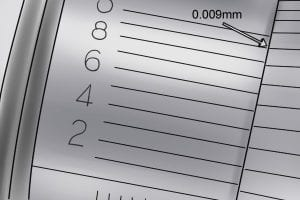Step 3 – Look at the Vernier Scale

If you have a vernier micrometer, you may next read the value indicated on the vernier scale (if you don’t have a vernier micrometer or don’t require the accuracy, go to Step 4).

The vernier scale of a metric micrometer has a measuring range of 0.01mm and is graduated in 10 increments. Each increment represents 0.001mm.

The reading on the vernier scale is the value that aligns best with any increment on the thimble scale (0.009mm in the image on the left).

Step 3 – Look at the Vernier Scale

If you have a vernier micrometer, you may next read the value indicated on the vernier scale (if you don’t have a vernier micrometer or don’t require the accuracy, go to Step 4).

The vernier scale of a metric micrometer has a measuring range of 0.01mm and is graduated in 10 increments. Each increment represents 0.001mm.

The reading on the vernier scale is the value that aligns best with any increment on the thimble scale (0.009mm in the image on the left).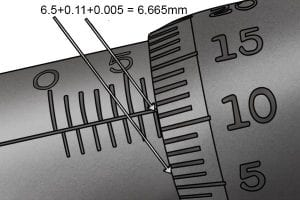Step 4 – Add Values Together

To get your total reading, add the values from each of the scales together (sleeve + thimble ( + vernier) = measured value).

e.g. 6.5mm + 0.11mm = 6.66mm.

Because the reading on the thimble scale fell roughly halfway between two values, we can estimate that the reading is in fact, 6.665mm.

A vernier scale, however, would provide the smallest part of the measurement (0.005mm in this case) more accurately.

Step 4 – Add Values Together

To get your total reading, add the values from each of the scales together (sleeve + thimble ( + vernier) = measured value).

e.g. 6.5mm + 0.11mm = 6.66mm.

Because the reading on the thimble scale fell roughly halfway between two values, we can estimate that the reading is in fact, 6.665mm.

A vernier scale, however, would provide the smallest part of the measurement (0.005mm in this case) more accurately.Call Now Button